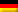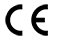TOP
•Deutsch

# Belt Tension Meter RTM-400##### Measuring range max. 10 - 800 Hz

Trummeter for measuring belt tension and for control the strand force of V-belts, tooth belts, power belts or similar. The optimal life time of a belt is depending on the correct belt tension.

The measured value is shown as frequency in Hz or strand force in N.

Non-contact measuring occurs by infrared light### Special Features

Measuring principle: Frequency measurement by LED light while the belt is not moving. The belt is toughed by hand to create vibration.

The readings can be displayed as frequency (Hz) or strand force (N or lbf)

The belt tension meter RTM-400 includes a display unit as well as 2 measuring probes:
– plug in probe for one-hand operation
– measuring probe with cable for limited access space

For determinating the belt force in Newton, 2 parameters are needed. Thereby the following restrictions are obtained:
– free strand length 9.99 m
– belt mass up to 9.999 kg/m

Belt tension meter with measuring range 10 – 800 Hz

Belt tension tester with multilingual operator guidance and display (German, English, French, Italian, Spanish, Portuguese, Swedish, Norwegian, Danish, Finnish)

Manufacturer`s calibration report is included

### Standard Features

Belt tension devise for easy and save operation

Trummeter with rugged, handy plastic housing

Microprocessor controlled belt tension meter

Belt tension tester for measurements with highest precision

### Available Models

 Model Measuring Range RTM-400 max. 10 – 800 Hz
Specifications

 Measuring range: 10 – 800 Hz Digital sampling error: < 1 % Indicator error: ±1 Hz Total error: < 5 % of measuring value Adjustable measuring units: Hz (Frequency) or N, lbf (Strand force) Measuring distance: 3 – 20 mm Display: 2 line LCD, 16 characters per line Languages: 10 languages (German, English, French, Italian, Spanish, Portuguese, Swedish, Norwegian, Danish, Finnish) Input ranges: Free strand length: up to 9.99 m Belt mass: up to 9.999 kg/m Temperature ranges: Operation: +10 °C … 50 °C, Transport: -5 °C … +50 °C Air humidity: 85 % RH, max. Power supply: 9 V – Battery Housing: Plastic (ABS) Dimensions: 126 x 80 x 37 (LxWxH) Weight net (gross): Approx. 170 g (approx. 660 g)

Specifications subject to change without notice!

Belt Masses

 Ribbed V-belts PJ = 0.082 PM = 1.100 PL = 0.320 kg/m per 10 ribs V-belts SPZ = 0.074 SPB = 0.195 10 = 0.06417 = 0.196 22 = 0.324 32 = 0.668 SPA = 0.123 SPC = 0.377 13 = 0.109 20 = 0.266 25 = 0.420 40 = 0.958 kg/m per belt Power belts SPZ = 0.120 SPB = 0.261 3V/9J = 0.120 8V/25J = 0.693 SPA = 0.166 SPC 0 0.555 5 V/15J = 0.252 kg/m per rib Polyurethane Toothed belts T 2.5 = 0.015 T 10 = 0.045 AT 3 0 0.023 AT 10 = 0.063 T 5 = 0.024 T 20 = 0.084 AT 5 = 0.034 AT 20 = 0.106 kg/m per 10 mm width

To measure the belt mass precisely, we recommend that you weigh the drive belt and then recalculate this weight based on a belt length of 1 meter.

The table above contains comparison values for some belts.

OperationThe belt tension can be measured only when the drive is stationary. Preferably, the belt tension should always be measured at the center of the longer belt strand between the two drive pulleys. The distance to the belt can be 3 – 20 mm. Depending upon ambient light and surface of belt, the measurement can occur from a distance of max. 60 mm, whereas darker light and better reflection (can be obtained by attaching a reflective tape) admit a larger measuring distance.

Measurement:
The belt is tapped in order to make it natural oscillating. This static natural frequency is then measured by the probe with the aid of pulsed light. Care must be taken to ensure the light is sufficiently reflected by the belt. A reflective tape must be fixed if necessary.

General information:
To measure the belt mass precisely, we recommend that you weigh the drive belt and then recalculate this weight based on a belt of 1 meter. The strand force is calculated using the formula:F = strand force in N m = linear belt mass in kg/m L = length of the free belt strand in m f = natural frequency of the free belt measured in Hz

Note
Measurement deviations of up to ±10 % for several measurements taken on the same drive belt are as a rule not caused by a measurement error or fault in the unit. In most cases, measurement deviations are due to the mechanical tolerances of the drive systems.

Attention!
Newton- or poundforce-calculations have a square factor higher error result!

Set point:
The set point for belt tension is specified as natural frequency in Hz or as strand force in N.
It is depending to the characteristics of the drive. Alternative the set point can be calculated with the formula:P = motor power in kW z = number of belts v = speed of belt = D x n/19100 D = effective diameter of the small pulley in mm n = revolution of the small pulley (Upm) m x v² = centrifugal force (relevant for revolutions > 800 Upm) m = weight of belt in kg/m according the table (for a belt)

Delivery Includes